### IMO Shortlist 1994 problem G3

Kvaliteta:
Avg: 0,0
Težina:
Avg: 7,0
A circle$C$ has two parallel tangents$L'$ and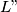$L"$. A circle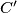$C'$ touches$L'$ at$A$ and$C$ at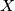$X$. A circle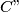$C"$ touches$L"$ at$B$,$C$ at$Y$ and$C'$ at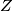$Z$. The lines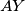$AY$ and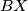$BX$ meet at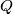$Q$. Show that$Q$ is the circumcenter of$XYZ$
Izvor: Međunarodna matematička olimpijada, shortlist 1994